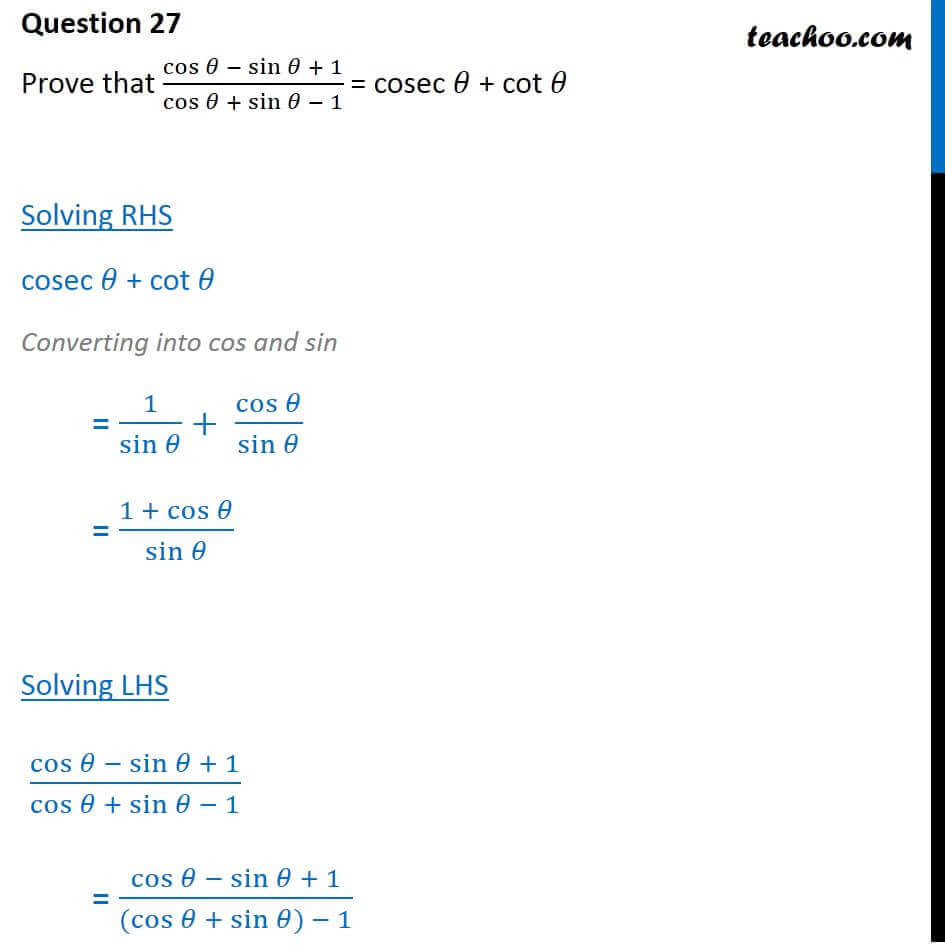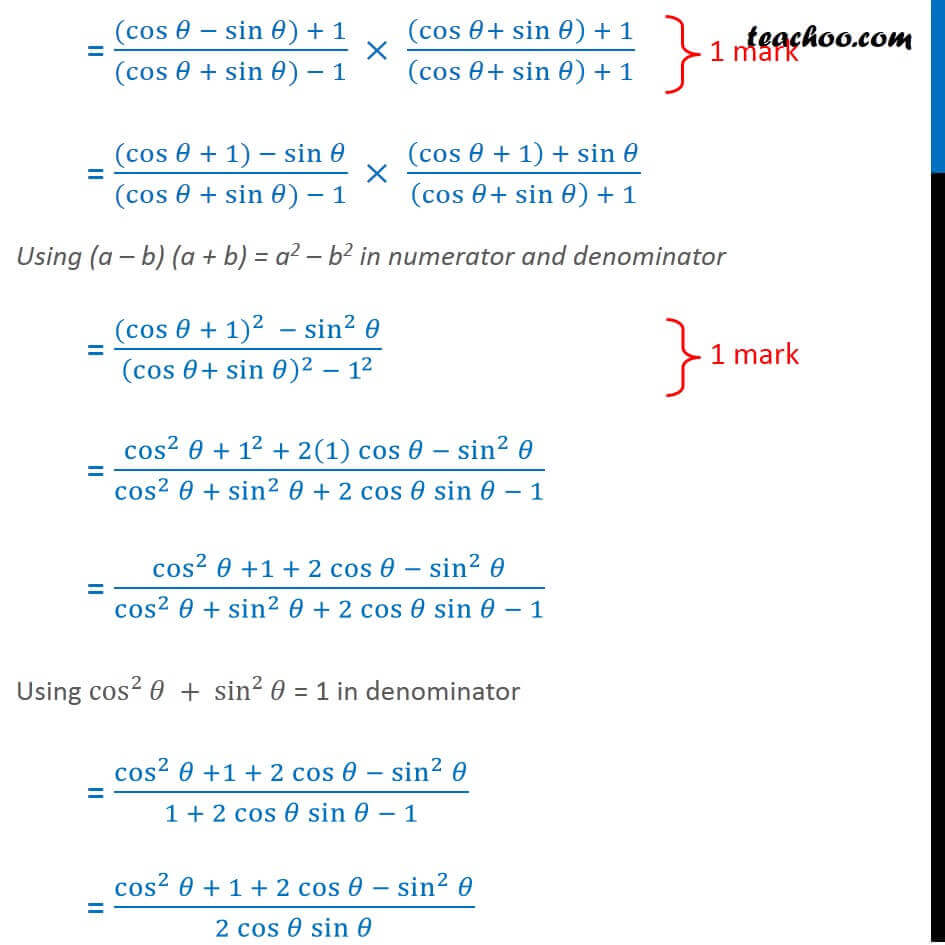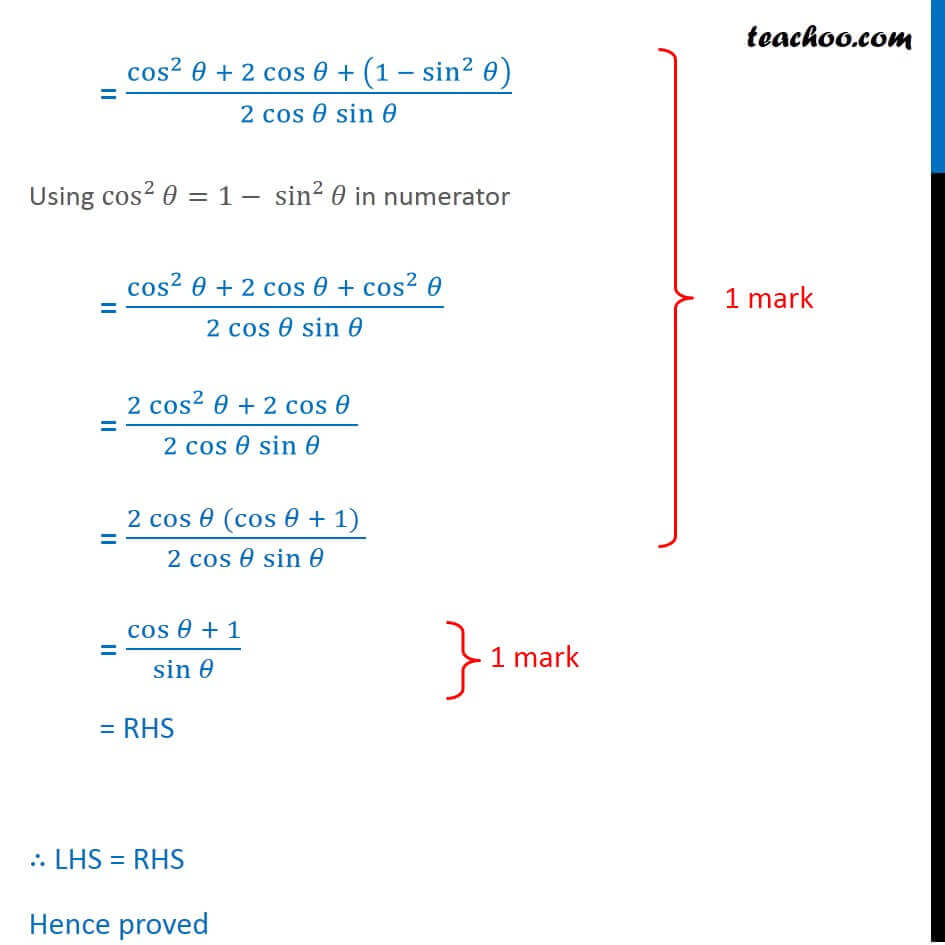CBSE Class 10 Sample Paper for 2018 Boards

Class 10
Solutions of Sample Papers for Class 10 Boards

### Prove that cos⁡ θ - sin⁡θ  + 1 /cos⁡ θ + sin⁡θ  - 1 = cosec θ + cot θ

This is a question of CBSE Sample Paper - Class 10 - 2017/18.Learn in your speed, with individual attention - Teachoo Maths 1-on-1 Class

### Transcript

Question 27 Prove that cos⁡〖𝜃 − sin⁡𝜃 + 1〗/cos⁡〖𝜃 + sin⁡𝜃 − 1〗 = cosec 𝜃 + cot 𝜃 Solving RHS cosec 𝜃 + cot 𝜃 Converting into cos and sin = 1/(sin 𝜃)+ cos⁡𝜃/sin⁡𝜃 = (1 + cos⁡𝜃)/sin⁡𝜃 Solving LHS cos⁡〖𝜃 − sin⁡𝜃 + 1〗/cos⁡〖𝜃 + sin⁡𝜃 − 1〗 = cos⁡〖𝜃 − sin⁡𝜃 + 1〗/((cos⁡〖𝜃 + sin⁡𝜃) − 1〗 ) Question 27 Prove that cos⁡〖𝜃 − sin⁡𝜃 + 1〗/cos⁡〖𝜃 + sin⁡𝜃 − 1〗 = cosec 𝜃 + cot 𝜃 Solving RHS cosec 𝜃 + cot 𝜃 Converting into cos and sin = 1/(sin 𝜃)+ cos⁡𝜃/sin⁡𝜃 = (1 + cos⁡𝜃)/sin⁡𝜃 Solving LHS cos⁡〖𝜃 − sin⁡𝜃 + 1〗/cos⁡〖𝜃 + sin⁡𝜃 − 1〗 = cos⁡〖𝜃 − sin⁡𝜃 + 1〗/((cos⁡〖𝜃 + sin⁡𝜃) − 1〗 ) = 〖(cos〗⁡〖𝜃 − sin⁡𝜃) + 1〗/((cos⁡〖𝜃 + sin⁡𝜃) − 1〗 ) × ((cos⁡𝜃+ sin⁡𝜃 ) + 1)/((cos⁡𝜃+ sin⁡𝜃 ) + 1) = (〖(cos〗⁡𝜃 + 1) − sin⁡𝜃)/((cos⁡〖𝜃 + sin⁡𝜃) − 1〗 ) × ((cos⁡𝜃 + 1) + sin⁡𝜃)/((cos⁡𝜃+ sin⁡𝜃 ) + 1) Using (a – b) (a + b) = a2 – b2 in numerator and denominator = (〖〖(cos〗⁡𝜃 + 1)〗^2 − sin^2⁡𝜃)/((cos⁡𝜃+ sin⁡𝜃 )^2 − 1^2 ) = (cos^2⁡𝜃 + 1^2 + 2(1) cos⁡𝜃 − sin^2⁡𝜃)/(cos^2⁡𝜃 + sin^2⁡𝜃 + 2 cos⁡𝜃 sin⁡𝜃 − 1) = (cos^2⁡𝜃 +1 + 2 cos⁡𝜃 − sin^2⁡𝜃)/(cos^2⁡𝜃 + sin^2⁡𝜃 + 2 cos⁡𝜃 sin⁡𝜃 − 1) Using cos^2⁡𝜃 + sin^2⁡𝜃 = 1 in denominator = (cos^2⁡𝜃 +1 + 2 cos⁡𝜃 − sin^2⁡𝜃)/(1 + 2 cos⁡𝜃 sin⁡𝜃 − 1) = (cos^2⁡𝜃 + 1 + 2 cos⁡𝜃 − sin^2⁡𝜃)/(2 cos⁡𝜃 sin⁡𝜃 ) = (cos^2⁡𝜃 + 2 cos⁡𝜃 + (1 − sin^2⁡𝜃 ))/(2 cos⁡𝜃 sin⁡𝜃 ) Using cos^2⁡𝜃=1− sin^2⁡𝜃 in numerator = (cos^2⁡𝜃 + 2 cos⁡𝜃 + cos^2⁡𝜃)/(2 cos⁡𝜃 sin⁡𝜃 ) = (2 cos^2⁡𝜃 + 2 cos⁡𝜃 )/(2 cos⁡𝜃 sin⁡𝜃 ) = (2 cos⁡𝜃 (cos⁡𝜃 + 1) )/(2 cos⁡𝜃 sin⁡𝜃 ) = (cos⁡𝜃 + 1)/sin⁡𝜃 = RHS ∴ LHS = RHS Hence proved